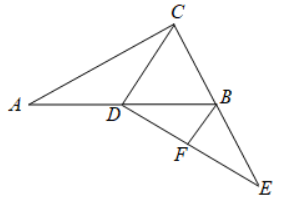$\text{A.}$ 5 $\text{B.}$ 4 $\text{C.}$ 6 $\text{D.}$ 8
【答案】 A

【解析】 解: $\because$ 在Rt $\triangle A B C$ 中， $\angle A C B=90^{\circ} ， A C=16 ， B C=12$ ，
$$\therefore A B=\sqrt{A C^2+B C^2}=\sqrt{16^2+12^2}=20 \text {. }$$

$$\therefore C D=\frac{1}{2} A B=10 \text {. }$$
$\because F$ 为 $D E$ 中点， $B E=B C$ 即点 $B$ 是 $E C$ 的中点，
$\therefore B F$ 是 $\triangle C D E$ 的中位线，则 $B F=\frac{1}{2} C D=5$.